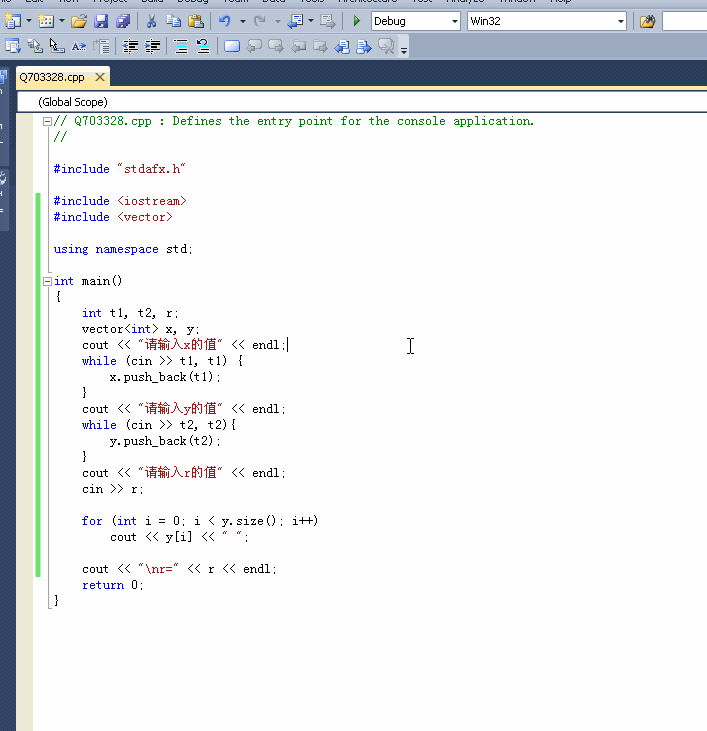2018-10-21 12:17

# C++新手，求大佬们帮一下。怎样才能连续输入？

cout << "请输入x的值" << endl;
while (cin >> t1) {
x.push_back(t1);
}
cout << "请输入y的值" << endl;
while (cin >> t2){
y.push_back(t2);
}
cout << "请输入r的值" << endl;
cin >> r;
`

why？

• 点赞
• 写回答
• 关注问题
• 收藏
• 复制链接分享
• 邀请回答

#### 3条回答

• 需要清除当前流的错误状态~

点赞 评论 复制链接分享
•回答这么多问题就耍赖把我的积分一笔勾销了 3年前
``````#include <iostream>
#include <vector>

using namespace std;

int main()
{
int t1, t2, r;
vector<int> x, y;
cout << "请输入x的值" << endl;
while (cin >> t1, t1) {
x.push_back(t1);
}
cout << "请输入y的值" << endl;
while (cin >> t2, t2){
y.push_back(t2);
}
cout << "请输入r的值" << endl;
cin >> r;

for (int i = 0; i < y.size(); i++)
cout << y[i] << " ";

cout << "\nr=" << r << endl;
return 0;
}
```````如果问题得到解决，麻烦点下我回答右边的采纳，谢谢`

点赞 评论 复制链接分享
• 你输入EOL结束第一个while循环后 cin对象会进入错误状态所以后续的输入会无效，解决方法 在两个while循环后都加上cin.clear()

点赞 评论 复制链接分享